# 《Python有什么好学的》之上下文管理器

“Python有什么好学的”这句话可不是反问句，而是问句哦。

## 常见的文件操作方式

``````def read_file_1():
f = open('file_demo.py', 'r')
try:
except Exception as e:
pass
f.close()
``````

1. 资源不释放，直到不可控的垃圾回收来了，甚至直到程序结束
2. 中间对文件修改时，修改的信息还没来得及写入文件
3. 整个代码显得不规范

## 使用上下文管理器

``````def read_file_2():
with open('file_demo.py', 'r') as f:
``````

## 实现上下文管理器

``````# 大概意思而已 with = open + do + close
def with():
open(xxxx)
doSomething(xxxx)
close(xxxx)
``````

``````from contextlib import contextmanager

@contextmanager
def c(s):
print(s + 'start')
yield s
print(s + 'end')
``````

“打印start”相当于open，而“打印end”相当于close，yield语法和修饰器（@）不熟悉的同学可以复习一下这些文章：生成器修饰器

``````def test_context():
with c('123') as cc:
print('in with')
print(type(cc))

if __name__ == '__main__':
test_context()
``````### 异常处理

``````def test_context():
with c('123') as cc:
print('in with')
print(type(cc))
raise Exception
````````````@contextmanager
def c():
print('start')
try:
yield
finally:
print('end')

def test_except():
try:
with c() as cc:
print('in with')
raise Exception

except:
print('catch except')
``````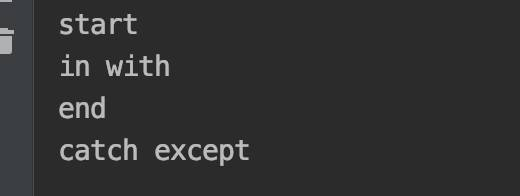## 实现上下文管理器类

``````class ContextClass(object):
def __init__(self, s):
self.s = s

def __enter__(self):
print(self.s + 'call enter')
return self

def __exit__(self, exc_type, exc_val, exc_tb):
print(self.s + 'call exit')

def test(self):
print(self.s + 'call test')
``````

``````def test_context():
with ContextClass('123') as c:
print('in with')
c.test()
print(type(c))
print(isinstance(c, ContextClass))

print('')
c = ContextClass('123')
print(type(c))
print(isinstance(c, ContextClass))

if __name__ == '__main__':
test_context()
``````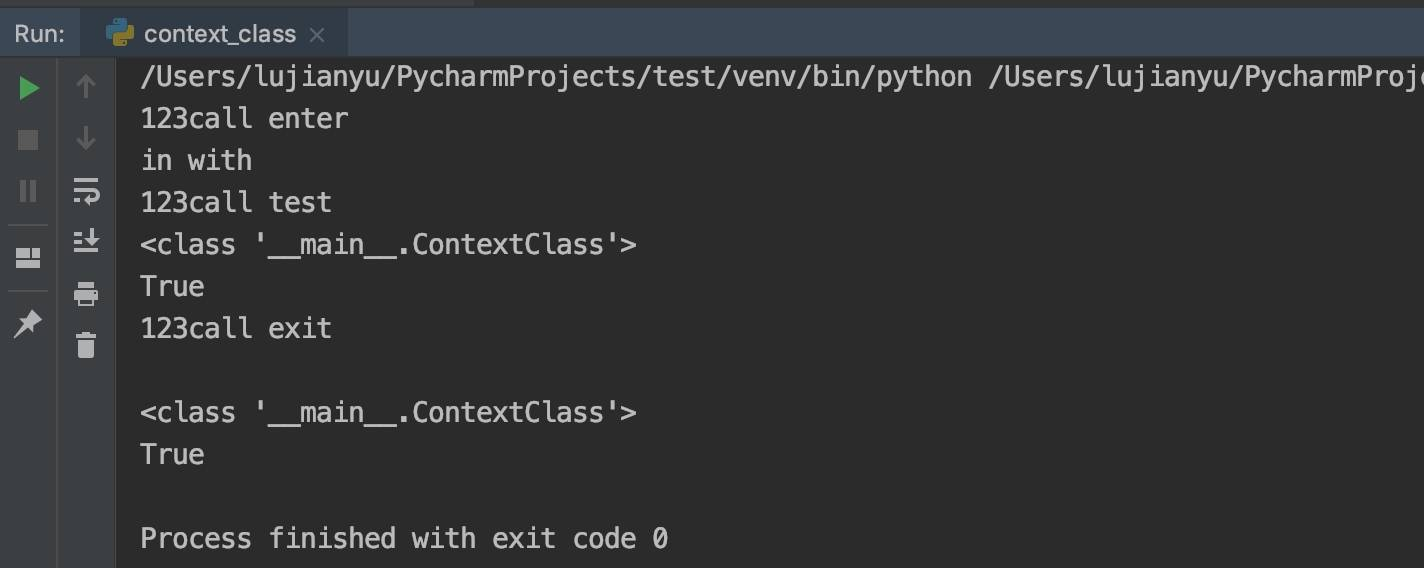### 异常处理

``````class ContextClass(object):
def __init__(self, s):
self.s = s

def __enter__(self):
print(self.s + 'call enter')
return self

def __exit__(self, exc_type, exc_val, exc_tb):
print(self.s + 'call exit')

class ContextExceptionClass(object):
def __init__(self, s):
self.s = s

def __enter__(self):
print(self.s + 'call enter')
return self

def __exit__(self, exc_type, exc_val, exc_tb):
print(self.s + 'call exit')
return True

def test_context():
with ContextExceptionClass('123') as c:
print('in with')
t = c.test()
print(type(t))

# with ContextClass('456') as c:
# print('in with')
# t = c.test()
# print(type(t))

if __name__ == '__main__':
test_context()
``````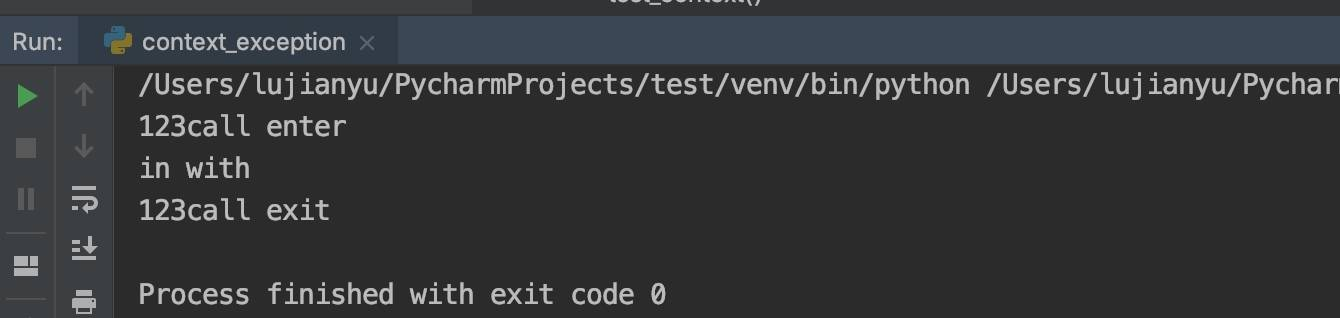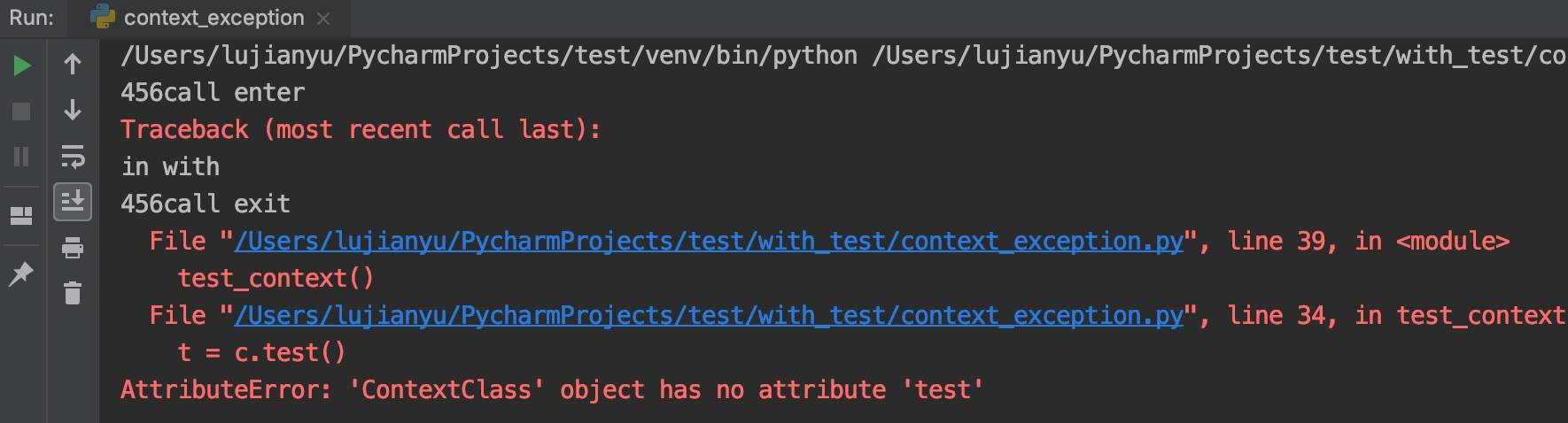``````class ContextExceptionClass(object):
def __init__(self, s):
self.s = s

def __enter__(self):
print(self.s + 'call enter')
return self

def __exit__(self, exc_type, exc_val, exc_tb):
print(self.s + 'call exit')
print(str(exc_type) + ' ' + str(exc_val) + ' ' + str(exc_tb))
return True
``````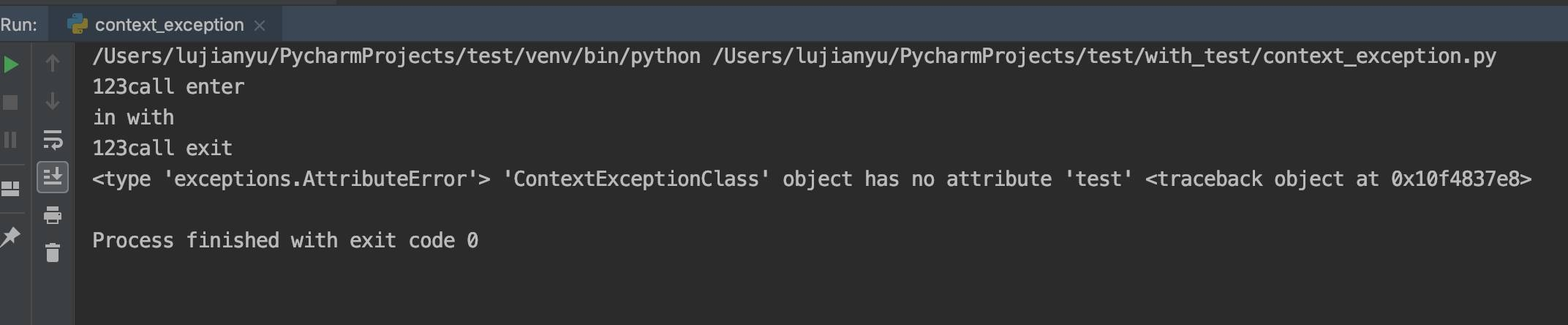Categories: Python特色文章